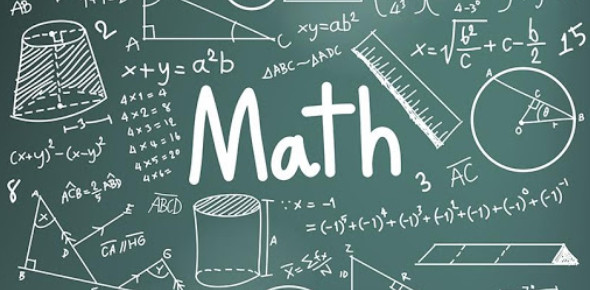# Random Math Quiz Questions And Answers

Reviewed by Janaisa Harris
Janaisa Harris, BA-Mathematics |
Mathematics Expert
Review Board Member
Ms. Janaisa Harris, an experienced educator, has devoted 4 years to teaching high school math and 6 years to tutoring. She is now broadening her educational impact by engaging in curriculum mapping for her county. This endeavor enriches her understanding of educational strategies and their implementation.
, BA-Mathematics
Approved & Edited by ProProfs Editorial Team
The editorial team at ProProfs Quizzes consists of a select group of subject experts, trivia writers, and quiz masters who have authored over 10,000 quizzes taken by more than 100 million users. This team includes our in-house seasoned quiz moderators and subject matter experts. Our editorial experts, spread across the world, are rigorously trained using our comprehensive guidelines to ensure that you receive the highest quality quizzes.
| By Imeikyo
I
Imeikyo
Community Contributor
Quizzes Created: 2 | Total Attempts: 11,547
Questions: 10 | Attempts: 10,564SettingsDo you like math? If you like solving random math questions, this quiz is for you. We have a few simple and easy questions in this random math quiz for you. It will help you check your knowledge, calculation as well as memory. It is a good and light brain exercise for you and everyone who takes this quiz. Just go for the quiz, choose your answers, and get the perfect score. All the best! You can share the quiz with everyone around you.

• 1.

### What is pi (3.14159265) to the power of pi?

• A.

36.4621596072

• B.

30.9591444

• C.

27.1234567

• D.

9.8596

A. 36.4621596072
Explanation
When you raise the mathematical constant pi (π), which is approximately 3.14159265, to the power of itself, you get an approximate value of 36.4621596072. This is a mathematical calculation and the result is an irrational number, meaning it cannot be expressed as a simple fraction and has an infinite number of decimal places.

Rate this question:

• 2.

### What is the square root of pi?

• A.

1.46803087

• B.

1.80003919

• C.

1.77245385

• D.

1.90932839

C. 1.77245385
Explanation
The square root of pi is approximately 1.77245385. This can be calculated by finding the square root of the value of pi, which is approximately 3.14159265. Taking the square root of this value gives us the answer of 1.77245385.

Rate this question:

• 3.

### 4(1+1)= ________

• A.

1

• B.

2

• C.

3

• D.

8

D. 8
Explanation
The given equation is 4(1+1). In order to solve it, we first need to simplify the expression inside the parentheses, which is 1+1. This equals 2. Then, we multiply 4 by 2, which gives us the final answer of 8.

Rate this question:

• 4.

### 1.1111111111 x 2.2222222222= _________

• A.

1.2222222222

• B.

2.1111111111

• C.

2.3134156

• D.

2.4691358

D. 2.4691358
Explanation
When multiplying two decimal numbers, you multiply the numbers as if they were whole numbers and then count the total number of decimal places in the original numbers. In this case, the original numbers have 10 decimal places combined (10 in the first number and 10 in the second number). So, when multiplying 1.1111111111 by 2.2222222222, the product will have 10 decimal places. The correct answer, 2.4691358, has 10 decimal places, which matches the explanation.

Rate this question:

• 5.

### If you have a cube with a volume of 73.560059, what is the length of the cube?

• A.

3.17

• B.

4.19

• C.

5.32

• D.

2.33

B. 4.19
Explanation
The length of a cube can be determined by finding the cube root of its volume. In this case, the cube has a volume of 73.560059. By taking the cube root of this value, we find that the length of the cube is 4.19.

Rate this question:

• 6.

### 1x0+1= __________.

• A.

1

• B.

2

• C.

3

• D.

4

A. 1
Explanation
The given equation is 1 multiplied by 0, which equals 0. Adding 1 to 0 gives a final result of 1.

Rate this question:

• 7.

### 3/33= __________

• A.

0.9999999999

• B.

0.0303030303

• C.

0.0909090909

• D.

0.3333333333

C. 0.0909090909
Explanation
When we divide 3 by 33, we get a recurring decimal pattern of 0.0909090909. This means that the decimal 0.0909090909 repeats infinitely. Therefore, it is the correct answer to the given equation 3/33.

Rate this question:

• 8.

### 2.82842712 i ________ by 2.82842712 i = -8

• A.

X

• B.

/

• C.

+

• D.

-

A. X
Explanation
The equation 2.82842712i multiplied by 2.82842712i equals -8 can be represented as 2.82842712i^2 = -8. To solve for i, we need to find the square root of -8. The square root of -8 is not a real number, but it can be expressed as 2.82842712i. Therefore, the correct answer is "x," indicating that i is equal to 2.82842712.

Rate this question:

• 9.

### 100+200+300+100+150x0+50+200+50 =?

• A.

100

• B.

0

• C.

1000

• D.

10000

C. 1000
Explanation
The given equation involves addition and multiplication operations. Starting from left to right, we add the numbers 100, 200, 300, 100, 0, 50, 200, and 50. This gives us a sum of 1000. Therefore, the answer to the equation is 1000.

Rate this question:

• 10.

### (-10)x(-10)=?

• A.

-20

• B.

-100

• C.

100

• D.

1000

C. 100
Explanation
When multiplying two negative numbers, the result is always positive. In this case, (-10) multiplied by (-10) equals 100.

Rate this question:

Janaisa Harris |BA-Mathematics |
Mathematics Expert
Ms. Janaisa Harris, an experienced educator, has devoted 4 years to teaching high school math and 6 years to tutoring. She is now broadening her educational impact by engaging in curriculum mapping for her county. This endeavor enriches her understanding of educational strategies and their implementation.

Related TopicsBack to top